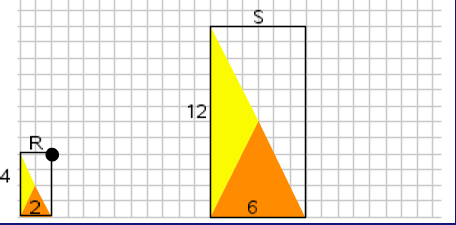# Ratios and Proportions / Ratios Within and Between Scaled Shapes

Activity 15 of 15
In this lesson, students use ratios to explore relationships that result from scaling figures and use reasoning to determine missing values for a given shape.

## Planning and Resources

Objectives
Students should understand the relationship between a scale factor and the corresponding sides of two figures. They recognize that area scales by the square of the linear scale factor of the sides of a figure.

Vocabulary
scale factor

Standard:

## Lesson Snapshot

#### Understanding

Students should recognize that lengths scale by a linear scale factor, but areas scale by the square of the scale factor that relates the lengths (if the area is measured in the unit of measurement derived from that used for length).

### What to look for

Have students examine the internal ratios between each shape as another way to make a connection of the ratio to scaling shapes.

### Sample Assessment

If the Rectangle S is an enlarged copy of Rectangle R, what is the scale factor?